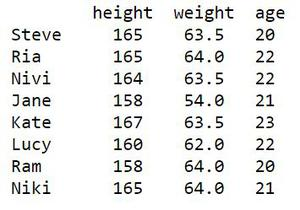# How to Count Distinct Values of a Pandas Dataframe Column?

• Last Updated : 19 Aug, 2020

Let’s see How to Count Distinct Values of a Pandas Dataframe Column?

Consider a tabular structure as given below which has to be created as Dataframe. The columns are height, weight and age. The records of 8 students form the rows.

Attention geek! Strengthen your foundations with the Python Programming Foundation Course and learn the basics.

To begin with, your interview preparations Enhance your Data Structures concepts with the Python DS Course. And to begin with your Machine Learning Journey, join the Machine Learning - Basic Level Course

First step is to create the Dataframe for the above tabulation. Look at the code snippet below.

## Python3

 `# import library``import` `pandas as pd`` ` `# create a Dataframe``df ``=` `pd.DataFrame({ ``  ``'height'` `: [``165``, ``165``, ``164``, ``              ``158``, ``167``, ``160``,``              ``158``, ``165``],``   ` `  ``'weight'` `: [``63.5``, ``64``, ``63.5``,``              ``54``, ``63.5``, ``62``,``              ``64``, ``64``],``   ` `  ``'age'` `: [``20``, ``22``, ``22``, ``           ``21``, ``23``, ``22``,``           ``20``, ``21``]},``   ` `   ``index ``=` `[``'Steve'``, ``'Ria'``, ``'Nivi'``, ``            ``'Jane'``, ``'Kate'``, ``'Lucy'``,``            ``'Ram'``, ``'Niki'``])`` ` `# show the Dataframe``df`

Output:Method 1: Using for loop.

The Dataframe has been created and one can hard coded using for loop and count the number of unique values in a specific column. For example In the above table, if one wishes to count the number of unique values in the column height. The idea is to use a variable cnt for storing the count and a list visited that has the previously visited values. Then for loop that iterates through the ‘height’ column and for each value, it checks whether the same value has already been visited in the visited list. If the value was not visited previously, then the count is incremented by 1.

Below is the implementation:

## Python3

 `# import library``import` `pandas as pd`` ` `# create a Dataframe``df ``=` `pd.DataFrame({ ``  ``'height'` `: [``165``, ``165``, ``164``, ``              ``158``, ``167``, ``160``,``              ``158``, ``165``],``   ` `  ``'weight'` `: [``63.5``, ``64``, ``63.5``,``              ``54``, ``63.5``, ``62``,``              ``64``, ``64``],``   ` `  ``'age'` `: [``20``, ``22``, ``22``, ``           ``21``, ``23``, ``22``,``           ``20``, ``21``]},``   ` `   ``index ``=` `[``'Steve'``, ``'Ria'``, ``'Nivi'``, ``            ``'Jane'``, ``'Kate'``, ``'Lucy'``,``            ``'Ram'``, ``'Niki'``])`` ` `# variable to hold the count``cnt ``=` `0`` ` `# list to hold visited values``visited ``=` `[]`` ` `# loop for counting the unique``# values in height``for` `i ``in` `range``(``0``, ``len``(df[``'height'``])):``   ` `    ``if` `df[``'height'``][i] ``not` `in` `visited: ``       ` `        ``visited.append(df[``'height'``][i])``         ` `        ``cnt ``+``=` `1`` ` `print``(``"No.of.unique values :"``,``      ``cnt)`` ` `print``(``"unique values :"``,``      ``visited)`

Output :

```No.of.unique values : 5
unique values : [165, 164, 158, 167, 160]
```

But this method is not so efficient when the Dataframe grows in size and contains thousands of rows and columns. To give an efficient there are three methods available which are listed below:

• pandas.unique()
• Dataframe.nunique()
• Series.value_counts()

Method 2: Using unique().

The unique method takes a 1-D array or Series as an input and returns a list of unique items in it. The return value is a NumPy array and the contents in it based on the input passed. If indices are supplied as input, then the return value will also be the indices of the unique value.

Syntax: pandas.unique(Series)

Example:

## Python3

 `# import library``import` `pandas as pd`` ` `# create a Dataframe``df ``=` `pd.DataFrame({ ``  ``'height'` `: [``165``, ``165``, ``164``, ``              ``158``, ``167``, ``160``,``              ``158``, ``165``],``   ` `  ``'weight'` `: [``63.5``, ``64``, ``63.5``,``              ``54``, ``63.5``, ``62``,``              ``64``, ``64``],``   ` `  ``'age'` `: [``20``, ``22``, ``22``, ``           ``21``, ``23``, ``22``,``           ``20``, ``21``]},``   ` `   ``index ``=` `[``'Steve'``, ``'Ria'``, ``'Nivi'``, ``            ``'Jane'``, ``'Kate'``, ``'Lucy'``,``            ``'Ram'``, ``'Niki'``])`` ` `# counting unique values``n ``=` `len``(pd.unique(df[``'height'``]))`` ` `print``(``"No.of.unique values :"``, ``      ``n)`

Output:

```No.of.unique values : 5
```

Method 3: Using Dataframe.nunique().

This method returns the count of unique values in the specified axis. The syntax is :

Syntax: Dataframe.nunique (axis=0/1, dropna=True/False)

Example:

## Python3

 `# import library``import` `pandas as pd`` ` `# create a Dataframe``df ``=` `pd.DataFrame({ ``  ``'height'` `: [``165``, ``165``, ``164``, ``              ``158``, ``167``, ``160``,``              ``158``, ``165``],``   ` `  ``'weight'` `: [``63.5``, ``64``, ``63.5``,``              ``54``, ``63.5``, ``62``,``              ``64``, ``64``],``   ` `  ``'age'` `: [``20``, ``22``, ``22``, ``           ``21``, ``23``, ``22``,``           ``20``, ``21``]},``   ` `   ``index ``=` `[``'Steve'``, ``'Ria'``, ``'Nivi'``, ``            ``'Jane'``, ``'Kate'``, ``'Lucy'``,``            ``'Ram'``, ``'Niki'``])`` ` `# check the values of ``# each row for each column``n ``=` `df.nunique(axis``=``0``)`` ` `print``(``"No.of.unique values in each column :\n"``,``      ``n)`

Output:

```No.of.unique values in each column :
height    5
weight    4
age       4
dtype: int64

```

To get the number of unique values in a specified column:

Syntax: Dataframe.col_name.nunique()

Example:

## Python3

 `# import library``import` `pandas as pd`` ` `# create a Dataframe``df ``=` `pd.DataFrame({ ``  ``'height'` `: [``165``, ``165``, ``164``, ``              ``158``, ``167``, ``160``,``              ``158``, ``165``],``   ` `  ``'weight'` `: [``63.5``, ``64``, ``63.5``,``              ``54``, ``63.5``, ``62``,``              ``64``, ``64``],``   ` `  ``'age'` `: [``20``, ``22``, ``22``, ``           ``21``, ``23``, ``22``,``           ``20``, ``21``]},``   ` `   ``index ``=` `[``'Steve'``, ``'Ria'``, ``'Nivi'``, ``            ``'Jane'``, ``'Kate'``, ``'Lucy'``,``            ``'Ram'``, ``'Niki'``])`` ` `# count no. of unique ``# values in height column``n ``=` `df.height.nunique()`` ` `print``(``"No.of.unique values in height column :"``,``      ``n)`

Output:

```No.of.unique values in height column : 5
```

Method 3: Using Series.value_counts().

This method returns the count of all unique values in the specified column.

Syntax: Series.value_counts(normalize=False, sort=True, ascending=False, bins=None, dropna=True)

Example:

## Python3

 `# import library``import` `pandas as pd`` ` `# create a Dataframe``df ``=` `pd.DataFrame({ ``  ``'height'` `: [``165``, ``165``, ``164``, ``              ``158``, ``167``, ``160``,``              ``158``, ``165``],``   ` `  ``'weight'` `: [``63.5``, ``64``, ``63.5``,``              ``54``, ``63.5``, ``62``,``              ``64``, ``64``],``   ` `  ``'age'` `: [``20``, ``22``, ``22``, ``           ``21``, ``23``, ``22``,``           ``20``, ``21``]},``   ` `   ``index ``=` `[``'Steve'``, ``'Ria'``, ``'Nivi'``, ``            ``'Jane'``, ``'Kate'``, ``'Lucy'``,``            ``'Ram'``, ``'Niki'``])`` ` ` ` `# getting the list of unique values``li ``=` `list``(df.height.value_counts())`` ` `# print the unique value counts``print``(``"No.of.unique values :"``,``      ``len``(li))`

Output:

```No.of.unique values : 5
```

My Personal Notes arrow_drop_up